Alan Horwitz

## Making Holes and Windows in SurfacesNBCDFPDF

In this article, we demonstrate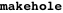, a program which removes points from any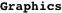or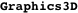picture whose coordinates satisfy a stated condition. We also demonstrate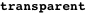and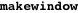, programs which make an entire or a specific portion of an opaque surface into a transparent mesh. We use these programs to view the region of integration for a triple integral. This article uses Mathematica 5.2, but with minor modifications all three programs work in Mathematica 6, as well as earlier versions. Theprogram duplicates some of the functionality of the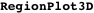command and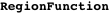option of the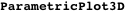command in Version 6, while theprogram behaves like the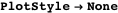option of the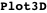command (see Editor’s Note for a demonstration of the Mathematica 6 code).

### Introduction

Given a 2D or 3D object, it is useful to view its cutaway image along an intersecting curve or surface. For example, we could cut an object along planes where,, orare constant by adjusting the PlotRange parameters. For cutting along a general curve or outside a surface, we introduce makehole, a program that removes vertices in Polygon, Line, and Point graphics primitives whose coordinates satisfy a stated condition (e.g., an equation, inequality, or compound inequality). We also introduce transparent and makewindow, which make an opaque surface into a transparent mesh by replacing each Polygon primitive with line segments around the boundary of the polygon. Viewing a region of integration for a triple integral in rectangular coordinates is one application of these programs.

### Making Surfaces Transparent

We first introduce transparent. To outline the boundary of a polygon, it repeats the first vertex at the end of each list of vertices and replaces all Polygon heads with Line. Unlike WireFrame in the Shapes package, transparent has adjustable PlotStyle options and works on graphics with either Graphics or Graphics3D heads.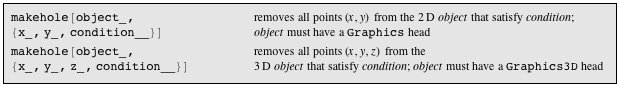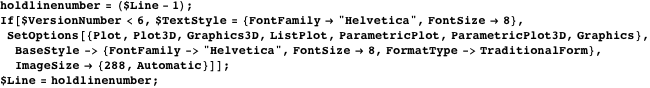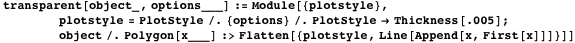We use Plot3D to draw the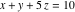plane and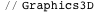to convert the SurfaceGraphics head to Graphics3D. Then we apply transparent and show the result.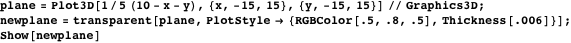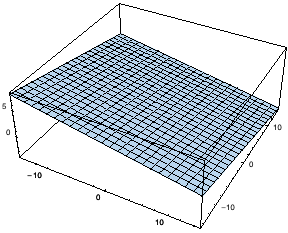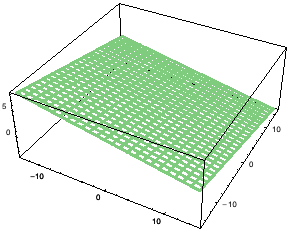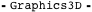### Making Holes

Before introducing makehole, we demonstrate its main ideas by removing portions of the following Graphics3D object that lie on or above theplane. The object consists of two polygons.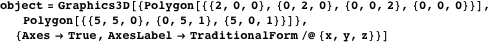The condition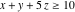is expressed as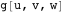in the following. Also,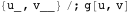is used to represent the vertices with either two or three coordinates that satisfy condition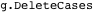removes vertices from the polygons that satisfy the condition, as well as polygons with no remaining vertices.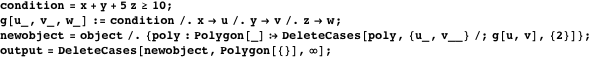We view the output in InputForm and observe that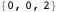and all vertices of the second polygon were removed from the original object because they satisfied.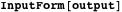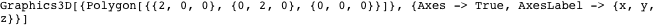We note that Polygon primitives with fewer than three remaining vertices will not be displayed by Show.

To demonstrate that this strategy works for more complicated objects, we generate a torus (suppressing the plot using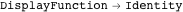), then use the same commands to remove the portions on and above.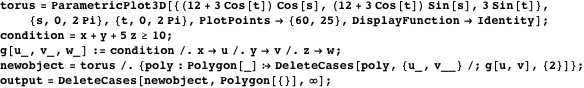We show the results with the transparentplane.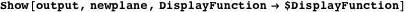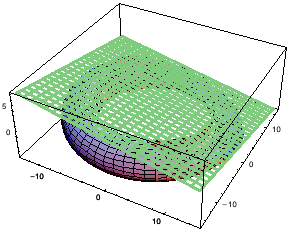The complete makehole program, which follows, removes vertices with two and three coordinates that satisfy a stated condition from within Polygon, Line, and Point primitives.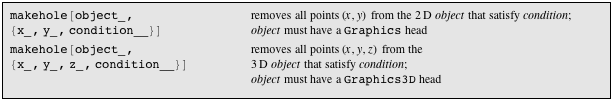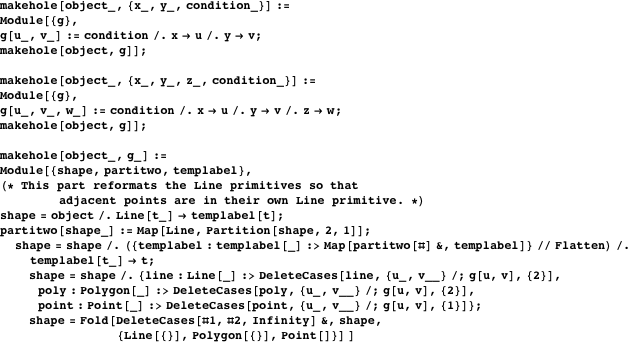To demonstrate makehole on graphics with Graphics heads, we use it to remove regions inside three ellipses from the graph of a spiral and three points. In order to remove a union of regions, we use || to represent “or,” so that points that satisfy any of the three inequalities are removed.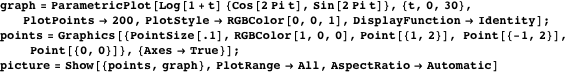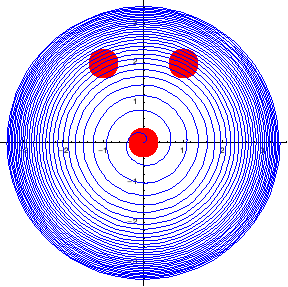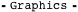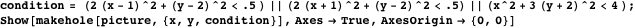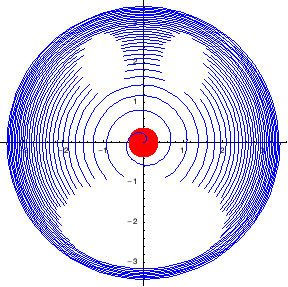### Making Windows in Surfaces

The makewindow program replaces Polygon with Line primitives if one of the vertices satisfies a stated condition.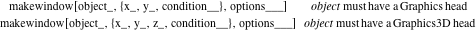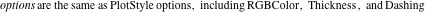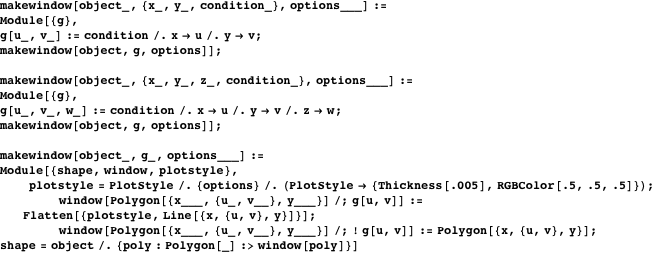To show how it works, we use the torus and the conditionto create a mesh window on portions lying above the corresponding plane. We use PlotStyle options to control the color and thickness of the mesh and to introduce dashing.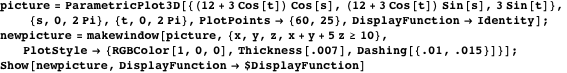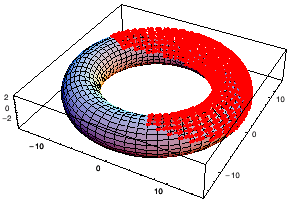### Showing the Region of Integration for a Triple Integral

We use makehole and makewindow to draw a region corresponding to the triple integral,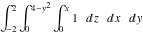. To visualize the region, we draw many of the bounding surfaces and then impose the three conditions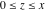,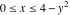, and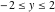from the limits of integration.

We first draw bounding surfaces for the region of integration. The surfaces drawn with Plot3D are converted to a Graphics3D head using. The blue bounding surface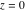and the purple surface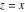are suppressed.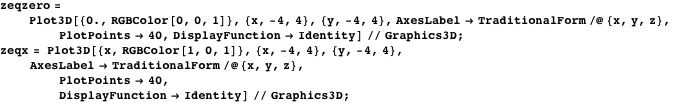To sketch the surface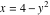, we view it as a parametrized surface between cross-sectional curves on planes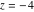and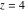. We color the surface red and suppress the plot.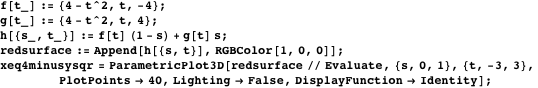The region of integration is enclosed by the surfaces. We omit the surfaces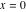,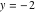, and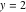from the image because the present region does not cross these planes.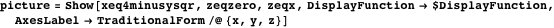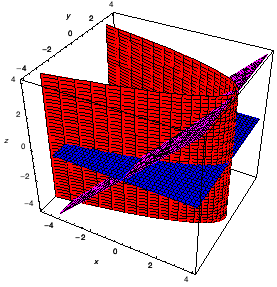To isolate the region of integration, we use makehole to remove all polygons that lie outside the region.

The condition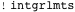removes all points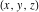whose coordinates do not satisfy each of,, and.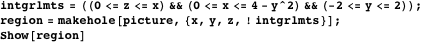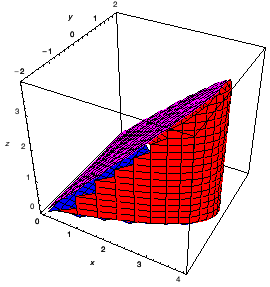To display the region inside the original image, we use makewindow to make everything outside the region transparent.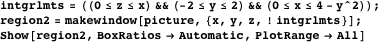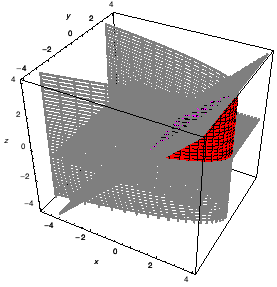### Conclusion

We have demonstrated programs for making holes and windows in portions of two- and three-dimensional objects which satisfy one or more specified inequalities. Applications include slicing an opaque surface along an intersecting surface and viewing the region of integration for a triple integral.

### Editor’s Note

This article describes techniques for working with legacy graphics in Mathematica. The code would need to be modified to work with GraphicsComplex and other extensions to the Mathematica graphics language in Version 6. Mathematica 6 also contains new features that overlap with the functionality described in this article.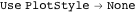to generate wireframe surfaces.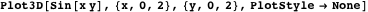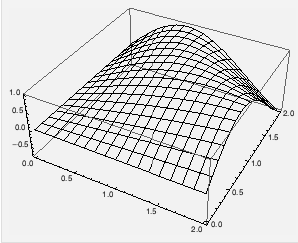Use Opacity to create partially transparent surfaces.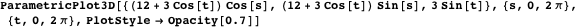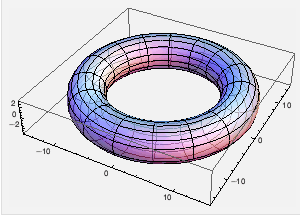Use RegionFunction to remove portions of a surface.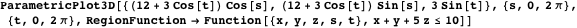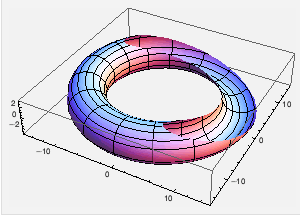Use RegionPlot3D to show a region defined by inequalities.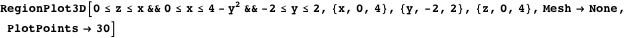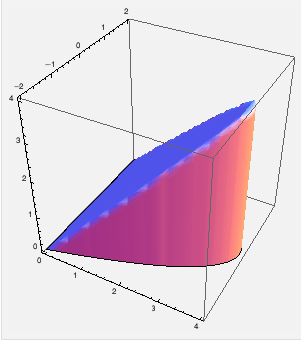### References

 A. Horwitz, “Making Holes and Windows in Surfaces,” The Mathematica Journal, 2011. dx.doi.org/doi:10.3888/tmj.10.4-4.